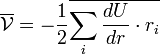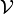# Virial theorem$\overline{ \mathcal{V} }= - \frac{1}{2} \overline{\sum_i \frac{dU}{dr}\cdot r_i}$
The overlines represent time averages. The right hand side is known as the virial of Clausius . (Note: Herbert Goldstein uses$T$ for the virial , however here we use$T$ for temperature, thus we use$\mathcal{V}$ as per Hansen and McDonald).